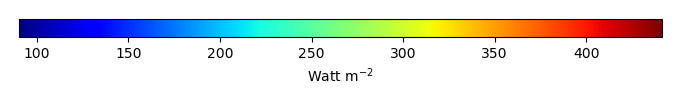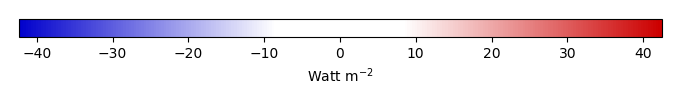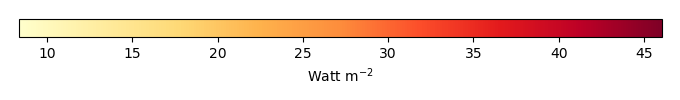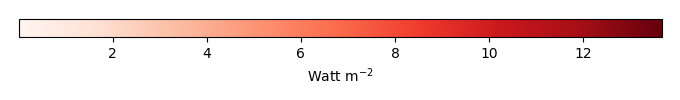# Mean State

Period Mean (original grids) [Watt m-2]
Model Period Mean (intersection) [Watt m-2]
Model Period Mean (complement) [Watt m-2]
Benchmark Period Mean (intersection) [Watt m-2]
Benchmark Period Mean (complement) [Watt m-2]
Bias [Watt m-2]
RMSE [Watt m-2]
Phase Shift [months]
Bias Score 
RMSE Score 
Seasonal Cycle Score 
Spatial Distribution Score 
Interannual Variability Score 
Overall Score 
Benchmark [-] 397.
CLM4 [-] 406. 406. 0.00 396. 408. 11.3 16.6 0.567 0.33 0.40 0.94 0.76 0.76 0.60
CLM4.5 [-] 406. 406. 0.00 396. 408. 11.3 16.6 0.567 0.33 0.40 0.94 0.76 0.76 0.60
CLM5 [-] 406. 406. 0.00 396. 408. 11.3 16.6 0.567 0.33 0.40 0.94 0.76 0.76 0.60
Period Mean (original grids) [Watt m-2]
Model Period Mean (intersection) [Watt m-2]
Model Period Mean (complement) [Watt m-2]
Benchmark Period Mean (intersection) [Watt m-2]
Benchmark Period Mean (complement) [Watt m-2]
Bias [Watt m-2]
RMSE [Watt m-2]
Phase Shift [months]
Bias Score 
RMSE Score 
Seasonal Cycle Score 
Spatial Distribution Score 
Interannual Variability Score 
Overall Score 
Benchmark [-] 384.
CLM4 [-] 395. 394. 0.00 384. 397. 10.5 17.7 0.930 0.61 0.63 0.89 0.83 0.74 0.72
CLM4.5 [-] 395. 394. 0.00 384. 397. 10.5 17.7 0.930 0.61 0.63 0.89 0.83 0.74 0.72
CLM5 [-] 395. 394. 0.00 384. 397. 10.5 17.7 0.930 0.61 0.63 0.89 0.83 0.74 0.72
Period Mean (original grids) [Watt m-2]
Model Period Mean (intersection) [Watt m-2]
Model Period Mean (complement) [Watt m-2]
Benchmark Period Mean (intersection) [Watt m-2]
Benchmark Period Mean (complement) [Watt m-2]
Bias [Watt m-2]
RMSE [Watt m-2]
Phase Shift [months]
Bias Score 
RMSE Score 
Seasonal Cycle Score 
Spatial Distribution Score 
Interannual Variability Score 
Overall Score 
Benchmark [-] 362.
CLM4 [-] 369. 368. 0.00 361. 382. 9.69 17.7 1.20 0.62 0.62 0.84 0.97 0.71 0.73
CLM4.5 [-] 369. 368. 0.00 361. 382. 9.69 17.7 1.20 0.62 0.62 0.84 0.97 0.71 0.73
CLM5 [-] 369. 368. 0.00 361. 382. 9.69 17.7 1.20 0.62 0.62 0.84 0.97 0.71 0.73
Period Mean (original grids) [Watt m-2]
Model Period Mean (intersection) [Watt m-2]
Model Period Mean (complement) [Watt m-2]
Benchmark Period Mean (intersection) [Watt m-2]
Benchmark Period Mean (complement) [Watt m-2]
Bias [Watt m-2]
RMSE [Watt m-2]
Phase Shift [months]
Bias Score 
RMSE Score 
Seasonal Cycle Score 
Spatial Distribution Score 
Interannual Variability Score 
Overall Score 
Benchmark [-] 239.
CLM4 [-] 205. 205. 0.00 238. 251. -30.9 33.7 0.0673 0.55 0.78 1.0 0.97 0.74 0.80
CLM4.5 [-] 205. 205. 0.00 238. 251. -30.9 33.7 0.0673 0.55 0.78 1.0 0.97 0.74 0.80
CLM5 [-] 205. 205. 0.00 238. 251. -30.9 33.7 0.0673 0.55 0.78 1.0 0.97 0.74 0.80
Period Mean (original grids) [Watt m-2]
Model Period Mean (intersection) [Watt m-2]
Model Period Mean (complement) [Watt m-2]
Benchmark Period Mean (intersection) [Watt m-2]
Benchmark Period Mean (complement) [Watt m-2]
Bias [Watt m-2]
RMSE [Watt m-2]
Phase Shift [months]
Bias Score 
RMSE Score 
Seasonal Cycle Score 
Spatial Distribution Score 
Interannual Variability Score 
Overall Score 
Benchmark [-] 411.
CLM4 [-] 427. 427. 0.00 410. 415. 19.7 22.9 1.86 0.070 0.33 0.72 0.57 0.70 0.45
CLM4.5 [-] 427. 427. 0.00 410. 415. 19.7 22.9 1.86 0.070 0.33 0.72 0.57 0.70 0.45
CLM5 [-] 427. 427. 0.00 410. 415. 19.7 22.9 1.86 0.070 0.33 0.72 0.57 0.70 0.45
Period Mean (original grids) [Watt m-2]
Model Period Mean (intersection) [Watt m-2]
Model Period Mean (complement) [Watt m-2]
Benchmark Period Mean (intersection) [Watt m-2]
Benchmark Period Mean (complement) [Watt m-2]
Bias [Watt m-2]
RMSE [Watt m-2]
Phase Shift [months]
Bias Score 
RMSE Score 
Seasonal Cycle Score 
Spatial Distribution Score 
Interannual Variability Score 
Overall Score 
Benchmark [-] 264.
CLM4 [-] 243. 243. 0.00 264. 277. -20.2 22.6 0.0306 0.70 0.84 1.0 0.98 0.86 0.87
CLM4.5 [-] 243. 243. 0.00 264. 277. -20.2 22.6 0.0306 0.70 0.84 1.0 0.98 0.86 0.87
CLM5 [-] 243. 243. 0.00 264. 277. -20.2 22.6 0.0306 0.70 0.84 1.0 0.98 0.86 0.87
Period Mean (original grids) [Watt m-2]
Model Period Mean (intersection) [Watt m-2]
Model Period Mean (complement) [Watt m-2]
Benchmark Period Mean (intersection) [Watt m-2]
Benchmark Period Mean (complement) [Watt m-2]
Bias [Watt m-2]
RMSE [Watt m-2]
Phase Shift [months]
Bias Score 
RMSE Score 
Seasonal Cycle Score 
Spatial Distribution Score 
Interannual Variability Score 
Overall Score 
Benchmark [-] 294.
CLM4 [-] 279. 278. 0.00 293. 336. -11.9 19.7 0.0697 0.76 0.78 0.99 0.99 0.83 0.85
CLM4.5 [-] 279. 278. 0.00 293. 336. -11.9 19.7 0.0697 0.76 0.78 0.99 0.99 0.83 0.85
CLM5 [-] 279. 278. 0.00 293. 336. -11.9 19.7 0.0697 0.76 0.78 0.99 0.99 0.83 0.85
Period Mean (original grids) [Watt m-2]
Model Period Mean (intersection) [Watt m-2]
Model Period Mean (complement) [Watt m-2]
Benchmark Period Mean (intersection) [Watt m-2]
Benchmark Period Mean (complement) [Watt m-2]
Bias [Watt m-2]
RMSE [Watt m-2]
Phase Shift [months]
Bias Score 
RMSE Score 
Seasonal Cycle Score 
Spatial Distribution Score 
Interannual Variability Score 
Overall Score 
Benchmark [-] 379.
CLM4 [-] 385. 385. 0.00 377. 410. 14.7 23.2 0.641 0.53 0.67 0.94 0.93 0.74 0.75
CLM4.5 [-] 385. 385. 0.00 377. 410. 14.7 23.2 0.641 0.53 0.67 0.94 0.93 0.74 0.75
CLM5 [-] 385. 385. 0.00 377. 410. 14.7 23.2 0.641 0.53 0.67 0.94 0.93 0.74 0.75
Period Mean (original grids) [Watt m-2]
Model Period Mean (intersection) [Watt m-2]
Model Period Mean (complement) [Watt m-2]
Benchmark Period Mean (intersection) [Watt m-2]
Benchmark Period Mean (complement) [Watt m-2]
Bias [Watt m-2]
RMSE [Watt m-2]
Phase Shift [months]
Bias Score 
RMSE Score 
Seasonal Cycle Score 
Spatial Distribution Score 
Interannual Variability Score 
Overall Score 
Benchmark [-] 244.
CLM4 [-] 214. 215. 0.00 244. 248. -28.8 31.6 0.00253 0.56 0.77 1.0 0.97 0.84 0.82
CLM4.5 [-] 214. 215. 0.00 244. 248. -28.8 31.6 0.00253 0.56 0.77 1.0 0.97 0.84 0.82
CLM5 [-] 214. 215. 0.00 244. 248. -28.8 31.6 0.00253 0.56 0.77 1.0 0.97 0.84 0.82
Period Mean (original grids) [Watt m-2]
Model Period Mean (intersection) [Watt m-2]
Model Period Mean (complement) [Watt m-2]
Benchmark Period Mean (intersection) [Watt m-2]
Benchmark Period Mean (complement) [Watt m-2]
Bias [Watt m-2]
RMSE [Watt m-2]
Phase Shift [months]
Bias Score 
RMSE Score 
Seasonal Cycle Score 
Spatial Distribution Score 
Interannual Variability Score 
Overall Score 
Benchmark [-] 351.
CLM4 [-] 353. 353. 0.00 350. 359. 3.02 14.9 0.381 0.75 0.72 0.97 0.88 0.71 0.79
CLM4.5 [-] 353. 353. 0.00 350. 359. 3.02 14.9 0.381 0.75 0.72 0.97 0.88 0.71 0.79
CLM5 [-] 353. 353. 0.00 350. 359. 3.02 14.9 0.381 0.75 0.72 0.97 0.88 0.71 0.79
Period Mean (original grids) [Watt m-2]
Model Period Mean (intersection) [Watt m-2]
Model Period Mean (complement) [Watt m-2]
Benchmark Period Mean (intersection) [Watt m-2]
Benchmark Period Mean (complement) [Watt m-2]
Bias [Watt m-2]
RMSE [Watt m-2]
Phase Shift [months]
Bias Score 
RMSE Score 
Seasonal Cycle Score 
Spatial Distribution Score 
Interannual Variability Score 
Overall Score 
Benchmark [-] 308.
CLM4 [-] 290. 290. 0.00 307. 324. -13.0 18.2 0.220 0.65 0.74 0.99 0.90 0.86 0.81
CLM4.5 [-] 290. 290. 0.00 307. 324. -13.0 18.2 0.220 0.65 0.74 0.99 0.90 0.86 0.81
CLM5 [-] 290. 290. 0.00 307. 324. -13.0 18.2 0.220 0.65 0.74 0.99 0.90 0.86 0.81
Period Mean (original grids) [Watt m-2]
Model Period Mean (intersection) [Watt m-2]
Model Period Mean (complement) [Watt m-2]
Benchmark Period Mean (intersection) [Watt m-2]
Benchmark Period Mean (complement) [Watt m-2]
Bias [Watt m-2]
RMSE [Watt m-2]
Phase Shift [months]
Bias Score 
RMSE Score 
Seasonal Cycle Score 
Spatial Distribution Score 
Interannual Variability Score 
Overall Score 
Benchmark [-] 344.
CLM4 [-] 305. 305. 0.00 311. 360. -2.78 21.3 0.550 0.59 0.63 0.93 0.99 0.73 0.75
CLM4.5 [-] 305. 305. 0.00 311. 360. -2.78 21.3 0.550 0.59 0.63 0.93 0.99 0.73 0.75
CLM5 [-] 305. 305. 0.00 311. 360. -2.78 21.3 0.550 0.59 0.63 0.93 0.99 0.73 0.75
Period Mean (original grids) [Watt m-2]
Model Period Mean (intersection) [Watt m-2]
Model Period Mean (complement) [Watt m-2]
Benchmark Period Mean (intersection) [Watt m-2]
Benchmark Period Mean (complement) [Watt m-2]
Bias [Watt m-2]
RMSE [Watt m-2]
Phase Shift [months]
Bias Score 
RMSE Score 
Seasonal Cycle Score 
Spatial Distribution Score 
Interannual Variability Score 
Overall Score 
Benchmark [-] 343.
CLM4 [-] 350. 350. 0.00 343. 360. 8.96 20.6 0.463 0.70 0.63 0.96 0.93 0.67 0.75
CLM4.5 [-] 350. 350. 0.00 343. 360. 8.96 20.6 0.463 0.70 0.63 0.96 0.93 0.67 0.75
CLM5 [-] 350. 350. 0.00 343. 360. 8.96 20.6 0.463 0.70 0.63 0.96 0.93 0.67 0.75
Period Mean (original grids) [Watt m-2]
Model Period Mean (intersection) [Watt m-2]
Model Period Mean (complement) [Watt m-2]
Benchmark Period Mean (intersection) [Watt m-2]
Benchmark Period Mean (complement) [Watt m-2]
Bias [Watt m-2]
RMSE [Watt m-2]
Phase Shift [months]
Bias Score 
RMSE Score 
Seasonal Cycle Score 
Spatial Distribution Score 
Interannual Variability Score 
Overall Score 
Benchmark [-] 244.
CLM4 [-] 216. 216. 0.00 243. 253. -27.1 29.3 0.0689 0.56 0.79 1.0 0.99 0.75 0.81
CLM4.5 [-] 216. 216. 0.00 243. 253. -27.1 29.3 0.0689 0.56 0.79 1.0 0.99 0.75 0.81
CLM5 [-] 216. 216. 0.00 243. 253. -27.1 29.3 0.0689 0.56 0.79 1.0 0.99 0.75 0.81
Period Mean (original grids) [Watt m-2]
Model Period Mean (intersection) [Watt m-2]
Model Period Mean (complement) [Watt m-2]
Benchmark Period Mean (intersection) [Watt m-2]
Benchmark Period Mean (complement) [Watt m-2]
Bias [Watt m-2]
RMSE [Watt m-2]
Phase Shift [months]
Bias Score 
RMSE Score 
Seasonal Cycle Score 
Spatial Distribution Score 
Interannual Variability Score 
Overall Score 
Benchmark [-] 374.
CLM4 [-] 372. 372. 0.00 369. 398. 13.3 21.8 0.734 0.45 0.59 0.92 0.93 0.77 0.71
CLM4.5 [-] 372. 372. 0.00 369. 398. 13.3 21.8 0.734 0.45 0.59 0.92 0.93 0.77 0.71
CLM5 [-] 372. 372. 0.00 369. 398. 13.3 21.8 0.734 0.45 0.59 0.92 0.93 0.77 0.71
Period Mean (original grids) [Watt m-2]
Model Period Mean (intersection) [Watt m-2]
Model Period Mean (complement) [Watt m-2]
Benchmark Period Mean (intersection) [Watt m-2]
Benchmark Period Mean (complement) [Watt m-2]
Bias [Watt m-2]
RMSE [Watt m-2]
Phase Shift [months]
Bias Score 
RMSE Score 
Seasonal Cycle Score 
Spatial Distribution Score 
Interannual Variability Score 
Overall Score 
Benchmark [-] 332.
CLM4 [-] 321. 321. 0.00 331. 370. -6.68 20.0 0.0934 0.79 0.68 0.99 0.91 0.89 0.82
CLM4.5 [-] 321. 321. 0.00 331. 370. -6.68 20.0 0.0934 0.79 0.68 0.99 0.91 0.89 0.82
CLM5 [-] 321. 321. 0.00 331. 370. -6.68 20.0 0.0934 0.79 0.68 0.99 0.91 0.89 0.82
Period Mean (original grids) [Watt m-2]
Model Period Mean (intersection) [Watt m-2]
Model Period Mean (complement) [Watt m-2]
Benchmark Period Mean (intersection) [Watt m-2]
Benchmark Period Mean (complement) [Watt m-2]
Bias [Watt m-2]
RMSE [Watt m-2]
Phase Shift [months]
Bias Score 
RMSE Score 
Seasonal Cycle Score 
Spatial Distribution Score 
Interannual Variability Score 
Overall Score 
Benchmark [-] 277.
CLM4 [-] 254. 254. 0.00 276. 298. -20.7 23.2 0.0749 0.62 0.79 0.99 0.99 0.91 0.85
CLM4.5 [-] 254. 254. 0.00 276. 298. -20.7 23.2 0.0749 0.62 0.79 0.99 0.99 0.91 0.85
CLM5 [-] 254. 254. 0.00 276. 298. -20.7 23.2 0.0749 0.62 0.79 0.99 0.99 0.91 0.85
Period Mean (original grids) [Watt m-2]
Model Period Mean (intersection) [Watt m-2]
Model Period Mean (complement) [Watt m-2]
Benchmark Period Mean (intersection) [Watt m-2]
Benchmark Period Mean (complement) [Watt m-2]
Bias [Watt m-2]
RMSE [Watt m-2]
Phase Shift [months]
Bias Score 
RMSE Score 
Seasonal Cycle Score 
Spatial Distribution Score 
Interannual Variability Score 
Overall Score 
Benchmark [-] 296.
CLM4 [-] 286. 288. 0.00 295. 324. -7.01 15.9 0.214 0.75 0.73 0.99 0.94 0.84 0.83
CLM4.5 [-] 286. 288. 0.00 295. 324. -7.01 15.9 0.214 0.75 0.73 0.99 0.94 0.84 0.83
CLM5 [-] 286. 287. 0.00 295. 324. -7.01 15.9 0.214 0.75 0.73 0.99 0.94 0.84 0.83
Period Mean (original grids) [Watt m-2]
Model Period Mean (intersection) [Watt m-2]
Model Period Mean (complement) [Watt m-2]
Benchmark Period Mean (intersection) [Watt m-2]
Benchmark Period Mean (complement) [Watt m-2]
Bias [Watt m-2]
RMSE [Watt m-2]
Phase Shift [months]
Bias Score 
RMSE Score 
Seasonal Cycle Score 
Spatial Distribution Score 
Interannual Variability Score 
Overall Score 
Benchmark [-] 406.
CLM4 [-] 424. 424. 0.00 405. 410. 18.6 23.4 2.09 0.15 0.37 0.67 0.72 0.75 0.50
CLM4.5 [-] 424. 424. 0.00 405. 410. 18.6 23.4 2.09 0.15 0.37 0.67 0.72 0.75 0.50
CLM5 [-] 424. 424. 0.00 405. 410. 18.6 23.4 2.09 0.15 0.37 0.67 0.72 0.75 0.50
Period Mean (original grids) [Watt m-2]
Model Period Mean (intersection) [Watt m-2]
Model Period Mean (complement) [Watt m-2]
Benchmark Period Mean (intersection) [Watt m-2]
Benchmark Period Mean (complement) [Watt m-2]
Bias [Watt m-2]
RMSE [Watt m-2]
Phase Shift [months]
Bias Score 
RMSE Score 
Seasonal Cycle Score 
Spatial Distribution Score 
Interannual Variability Score 
Overall Score 
Benchmark [-] 376.
CLM4 [-] 384. 383. 0.00 376. 374. 8.34 16.3 1.28 0.59 0.60 0.81 0.91 0.84 0.72
CLM4.5 [-] 384. 383. 0.00 376. 374. 8.34 16.3 1.28 0.59 0.60 0.81 0.91 0.84 0.72
CLM5 [-] 384. 383. 0.00 376. 374. 8.34 16.3 1.28 0.59 0.60 0.81 0.91 0.84 0.72
Period Mean (original grids) [Watt m-2]
Model Period Mean (intersection) [Watt m-2]
Model Period Mean (complement) [Watt m-2]
Benchmark Period Mean (intersection) [Watt m-2]
Benchmark Period Mean (complement) [Watt m-2]
Bias [Watt m-2]
RMSE [Watt m-2]
Phase Shift [months]
Bias Score 
RMSE Score 
Seasonal Cycle Score 
Spatial Distribution Score 
Interannual Variability Score 
Overall Score 
Benchmark [-] 307.
CLM4 [-] 289. 288. 0.00 303. 340. -11.5 22.3 0.489 0.46 0.57 0.96 0.99 0.70 0.71
CLM4.5 [-] 289. 288. 0.00 303. 340. -11.5 22.3 0.489 0.46 0.57 0.96 0.99 0.70 0.71
CLM5 [-] 289. 288. 0.00 303. 340. -11.5 22.3 0.489 0.46 0.57 0.96 0.99 0.70 0.71

# Temporally integrated period mean

BENCHMARK MEANMODEL MEANBIASBIAS SCORERMSERMSE SCOREBENCHMARK INTERANNUAL VARIABILITYMODEL INTERANNUAL VARIABILITYINTERANNUAL VARIABILITY SCOREBENCHMARK MAX MONTHMODEL MAX MONTHDIFFERENCE IN MAX MONTHSEASONAL CYCLE SCORESPATIAL TAYLOR DIAGRAMMODEL COLORS# Spatially integrated regional mean

MODEL COLORSREGIONAL MEANANNUAL CYCLEMONTHLY ANOMALYANNUAL CYCLE# All Models

BenchmarkCLM4CLM4.5CLM5# Data Information

creation_date: Mon Jun 30 23:41:07 PDT 2014

source_file: This product is generated from monthly 1 degree GEWEX SRB Radiation observations